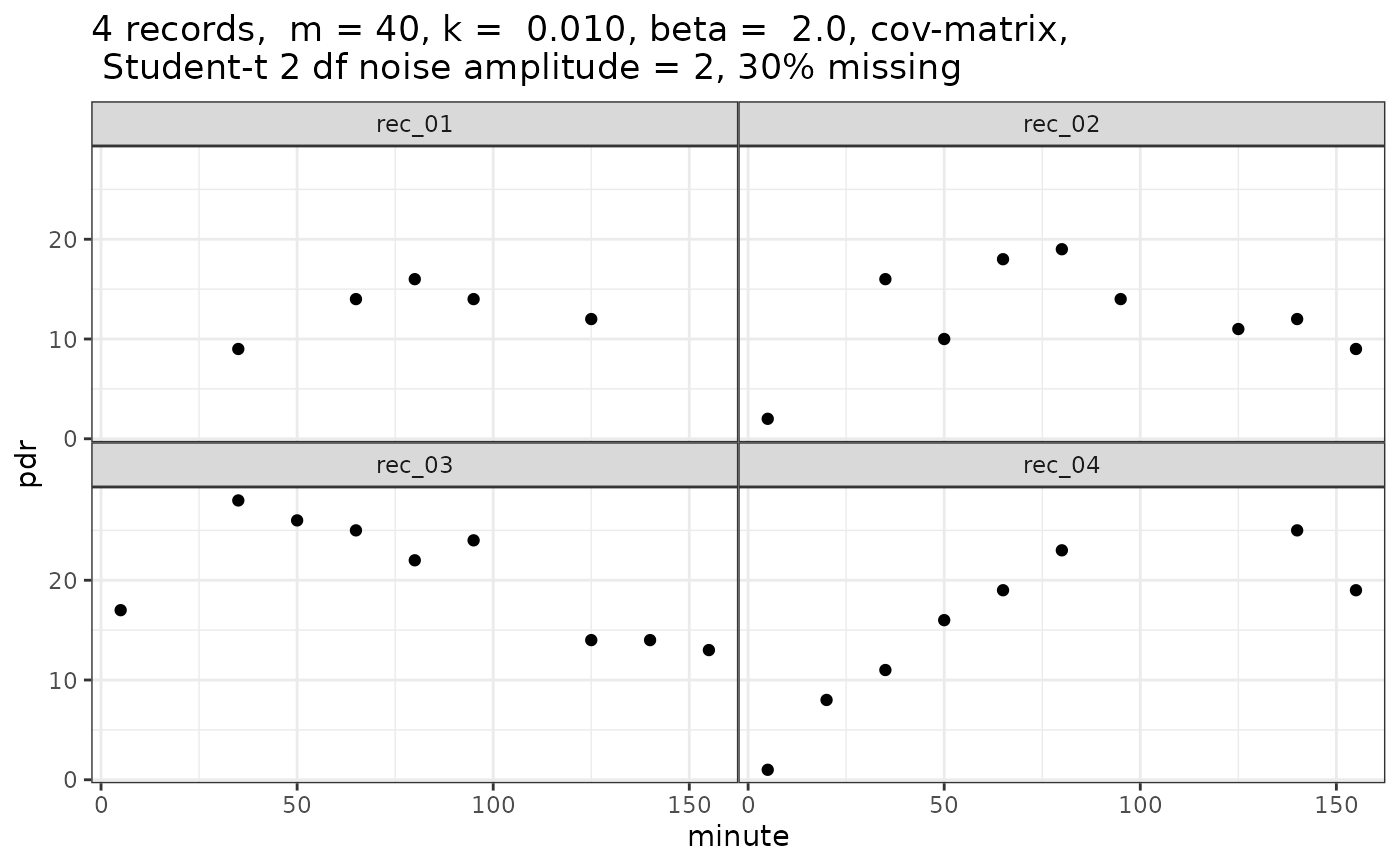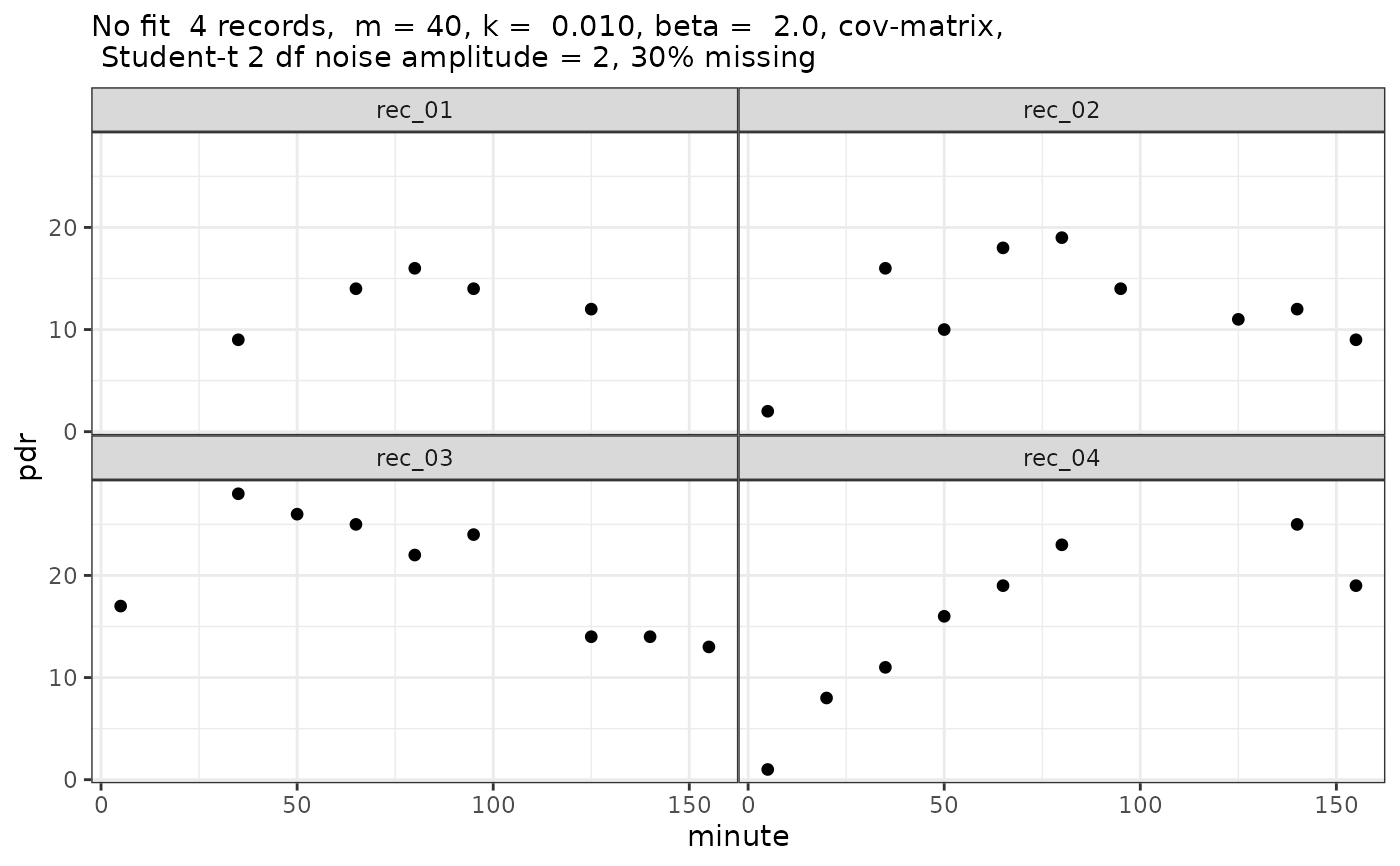Generates simulated breath test data, optionally with errors. If none of the three standard deviations m_std, k_std, beta_std is given, an empirical covariance matrix from USZ breath test data is used. If any of the standard deviations is given, default values for the others will be used.

## Usage

simulate_breathtest_data(
n_records = 10,
m_mean = 40,
m_std = NULL,
k_mean = 0.01,
k_std = NULL,
beta_mean = 2,
beta_std = NULL,
noise = 1,
cov = NULL,
student_t_df = NULL,
missing = 0,
seed = NULL,
dose = 100,
first_minute = 5,
step_minute = 15,
max_minute = 155
)

## Arguments

n_records

Number of records

m_mean, m_std

Mean and between-record standard deviation of parameter m giving metabolized fraction.

k_mean, k_std

Mean and between-record standard deviation of parameter k, in units of 1/minutes.

beta_mean, beta_std

Mean and between-record standard deviations of lag parameter beta

noise

Standard deviation of normal noise when student_t_df = NULL; scaling of noise when student_t_df >= 2.

cov

Covariance matrix, default NULL, i.e. not used. If given, overrides standard deviation settings.

student_t_df

When NULL (default), Gaussian noise is added; when >= 2, Student_t distributed noise is added, which generates more realistic outliers. Values from 2 to 5 are useful, when higher values are used the result comes close to that of Gaussian noise. Values below 2 are truncated to 2.

missing

When 0 (default), all curves have the same number of data points. When > 0, this is the fraction of points that were removed randomly to simulate missing

seed

Optional seed; not set if seed = NULL (default)

dose

Octanoate/acetate dose, almost always 100 mg, which is also the default

first_minute

First sampling time. Do not use 0 here, some algorithms do not converge when data near 0 are passed.

step_minute

Inter-sample interval for breath test

max_minute

Maximal time in minutes.

## Value

A list of class simulated_breathtest_data with 2 elements:

record

Data frame with columns patient_id(chr), m, k, beta, t50 giving the effective parameters for the individual patient record.

data

Data frame with columns patient_id(chr), minute(dbl), pdr(dbl) giving the time series and grouping parameters.

A comment is attached to the return value that can be used as a title for plotting.

## Examples

library(ggplot2)
pdr = simulate_breathtest_data(n_records = 4, seed = 4711, missing = 0.3,
student_t_df = 2, noise = 1.5) # Strong outliers
#
str(pdr, 1)
#> List of 2
#>  $record:'data.frame': 4 obs. of 5 variables: #> ..- attr(*, "cov")= num [1:3, 1:3] 1.88e+02 -2.60e-02 -2.04 -2.60e-02 7.74e-06 4.77e-04 -2.04 4.77e-04 1.82e-01 #> .. ..- attr(*, "dimnames")=List of 2 #>$ data  : tibble [31 × 3] (S3: tbl_df/tbl/data.frame)
#>   ..- attr(*, "comment")= chr "4 records,  m = 40, k =  0.010, beta =  2.0, cov-matrix, \n Student-t 2 df noise amplitude = 2, 30% missing"
#>  - attr(*, "class")= chr [1:2] "simulated_breathtest_data" "list"
#
pdr$record # The "correct" parameters #> patient_id m k beta t50_maes_ghoos #> 1 rec_01 30 0.0086 2.0 142 #> 2 rec_02 27 0.0140 2.4 100 #> 3 rec_03 46 0.0098 1.4 97 #> 4 rec_04 56 0.0076 2.2 169 # # Explicit plotting ggplot(pdr$data, aes(x = minute, y = pdr)) + geom_point() +
facet_wrap(~patient_id) + ggtitle(comment(pdr$data))# # Or use cleanup_data and null_fit for S3 plotting plot(null_fit(cleanup_data(pdr$data)))